# Let's sort with JavaScript 🔢

There are many different sorting algorithms out there such as quick sort, merge sort, insertion sort, bubble sort, etc., that could be useful in our day to day life, writing code which gets shipped to production. Knowing all of them is not necessary, but if you have a basic understanding of each one, you can decide on the most efficient one for your scenario.

## Introduction

Choosing a suboptimal sort algorithm could lead to longer completion time, code complexity, or worse, a program that crashes half way through an operation.

We use sorting algorithms everyday, `Array.sort` is one of the sort algorithms which is used to sort an array in ascending order. But that is not a solution for every scenario.

When choosing a sorting algorithm, we need to consider the complexity, or the number of operations performed (usually mentioned as `O(x)`, which is read Big O of x) and also number of swaps along the way. So let’s review and implement some of the most used ones together and learn about their complexity.

## Bubble sort

The way bubble sort works is very simple. You compare the first item of the collection with the second. If the first one is bigger, then swap the two. If not, move to the second item, and repeat the same. We keep repeating this until we reach the end of the list.

So far we have bubbled up the biggest item of the list to the far right side in its position. Now we repeat this for the rest of the items again until the list is sorted.

Let’s see this in action: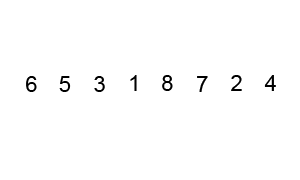``````function bubbleSort(list) {
let len = list.length;

for(let i = len - 1; i >= 0; i--) {
for(let j = 1; j <= i; j++) {
if(list[j - 1] > list[j]) {
let temp = list[j - 1];
list[j - 1] = list[j];
list[j] = temp;
}
}
}

return list;
}

bubbleSort([7, 5, 2, 3, 9, 6]); // [2, 3, 5, 6, 7, 9]``````

As you can see this algorithm is not optimal, in fact it is one of the heaviest in terms of number of operations in worst-case scenarios. But in terms of swaps, it’s one of the best since it sorts in place.

The complexity of bubble sort in worst-case is O(n2), read as `big O of n square`, where `n` is the number of items in the collection.

However, in a best case scenario (already sorted collections), it will be O(n) with O(1) swaps.

Case Complexity
Worst-case performance O(n2) comparisons
O(n2) swaps
Best-case performance O(n) comparisons
O(1) swaps
Average-case performance O(n2) comparisons
O(n2) swaps

## Selection sort

Selection sort is really simple like bubble sort. We go through the list, find the index of the lowest element, then swap the lowest element with the first one. Now that the first item is sorted, we repeat this for all remaining elements.

Let’s see this in action: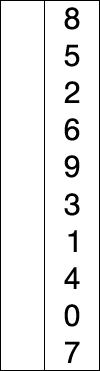``````function selectionSort(list) {
let minIndex, temp,
len = list.length;

for(let i = 0; i < len; i++) {
minIndex = i;
for(let j = i+1; j < len; j++) {
if(list[j] < list[minIndex]) {
minIndex = j;
}
}

temp = list[i];
list[i] = list[minIndex];
list[minIndex] = temp;
}

return list;
}

selectionSort([11, 25, 12, 22, 64]); //[11, 12, 22, 25, 64]``````

Let’s see how the list is sorted in each iteration in the above example:

Sorted list Unsorted sublist Lowest elements
[] [11, 25, 12, 22, 64] 11
 [25, 12, 22, 64] 12
[11, 12] [25, 22, 64] 22
[11, 12, 22] [25, 64] 25
[11, 12, 22, 25]  64
[11, 12, 22, 25, 64] []

In terms of complexity, this algorithm remains the same regardless of which scenario we’re facing. Which is O(n2) for comparisons, and O(n) swaps. But if you look at the code, it’s self explanatory and simple and sometimes we just want exactly that. In terms or swaps, it’s less than bubble sort.

Case Complexity
Worst-case performance O(n2) comparisons
O(n) swaps
Best-case performance O(n2) comparisons
O(n) swaps
Average-case performance O(n2) comparisons
O(n) swaps

## Insertion sort

This is like when I play cards and someone is handing me the them one by one. I usually put them in my hand in order as I receive them. Insertion sort builds the final list one item at a time. This means it’s less efficient for large lists relative to it’s competitors such as quick sort, or merge sort.

• Simple implementation (we’ll get there shortly).
• Efficient for small data sets.
• More efficient than bubble or selection sorts.
• In place.
• Online, can sort a list as it receives it.

Let’s see how it works in action: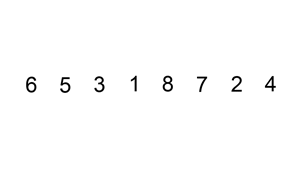``````function insertionSort(list){
let i, len = list.length, item, j;

for(i = 1; i < len; i++){
item = list[i];
j = i;

while(j > 0 && list[j-1] > item) {
list[j] = list[j-1];
j--;
}

list[j] = item;
}

return list;
}``````

In terms of complexity, it’s similar to bubble sort in worst and average cases with O(n2) for both comparisons and swaps. But in best case, it’s really efficient with O(n) comparisons and O(1) swaps.

Case Complexity
Worst-case performance O(n2) comparisons
O(n2) swaps
Best-case performance O(n) comparisons
O(1) swaps
Average-case performance O(n2) comparisons
O(n2) swaps

## Merge sort

Merge sort is in the divide and conquer algorithms and is implemented with a recursive pattern. We break down the list into small pieces until you have one item in each piece. Then we merge them back together but will compare them and put the items in order.

It’s really easy to understand, but let’s see it in action: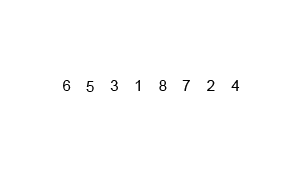``````function mergeSort(list) {
let len = list.length;
if(len < 2)
return list;
let mid = Math.floor(len/2),
left = list.slice(0,mid),
right =list.slice(mid);

return merge(mergeSort(left),mergeSort(right));
}

function merge(left, right) {
let result = [],
lLen = left.length,
rLen = right.length,
l = 0,
r = 0;
while(l < lLen && r < rLen) {
if(left[l] < right[r]) {
result.push(left[l++]);
}
else{
result.push(right[r++]);
}
}

return result.concat(left.slice(l)).concat(right.slice(r));
}``````

Merge sort is much better in terms of complexity from previous algorithms. It takes O(n log n) operations to sort an array. In terms of memory needed, it’s O(n) total with O(n) auxiliary if we use array and O(1) if we use a linked list.

Case Complexity
Worst-case performance O(n log n)
Best-case performance O(n log n)
Average-case performance O(n log n)
Worst-case space O(n) total, O(n) auxiliary with list, O(1) with linked list

## Quick sort

Quick sort is similar to merge sort, with the difference that the we don’t split the collection in half. We choose a pivot point and split from there. Once we have chosen the pivot point, we put all smaller items to the left and all the larger items to the right of that.

That means that the pivot point itself is sorted now. We continue this for left and right side recursively until we have the full list sorted.

Choosing the pivot could be random, middle point, first, or last item of the list. There are many ways to do this each with their own pros and cons.

Let’s see this in action to better understand the difference: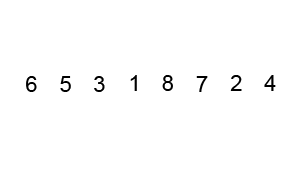``````function quickSort(list, left, right) {
let len = list.length,
pivot,
partitionIndex;

if(left < right) {
pivot = right;
partitionIndex = partition(list, pivot, left, right);

//sort left and right
quickSort(list, left, partitionIndex - 1);
quickSort(list, partitionIndex + 1, right);
}
return list;
}

function partition(list, pivot, left, right) {
let pivotValue = list[pivot],
partitionIndex = left;

for(let i = left; i < right; i++) {
if(list[i] < pivotValue) {
swap(list, i, partitionIndex);
partitionIndex++;
}
}
swap(list, right, partitionIndex);
return partitionIndex;
}

function swap(list, i, j) {
let temp = list[i];
list[i] = list[j];
list[j] = temp;
}

quickSort([11,8,14,3,6,2,7],0,6);
//[2, 3, 6, 7, 8, 11, 14]``````

As you can see, the more efficient the algorithm gets, the more complex the implementation will be. In terms of complexity, it’s worst than merge sort in worst-case, and equal in average and best.

Case Complexity
Worst-case performance O(n2)
Best-case performance O(n log n) with simple partition, O(n) with three-way partition
Average-case performance O(n log n)
Worst-case space O(n) auxiliary

## Heap sort

Heap sort is a comparison based sort, you can think of it as an improved version of selection sort. It divides it’s input into a sorted and an unsorted region, then iteratively shrinks the unsorted region by extracting the largest item and inserting it into the sorted region.

The unsorted region is kept in heap data structure to more quickly find the largest item in each step.

💡 Since there is no heap data structure in JavaScript, we can use an array to represent it.

That was a mouthful, so let’s see it in action: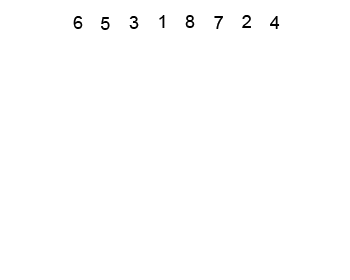``````function heapSort(list) {
let len = list.length;
let i = Math.floor(len / 2 - 1);
let j = len - 1;

while(i >= 0) {
heapify(list, len, i);

i--;
}

while(k >= 0) {
[list, list[k]] = [list[k], list];

heapify(list, k, 0);

k--;
}

return list;
}

function heapify(list, len, i){
let largest = i;
let left = i * 2 + 1;
let right = left + 1;

if(left < len && > list[left] > list[largest]) {
largest = left;
}

if(right < len && list[right] > list[largest]) {
largest = right;
}

if(largest != i) {
[list[i], list[largest]] = [list[largest], list[i]];

heapify(list, len, largest);
}

return list;
}``````

In the above code snippet, `heapify` function compares three elements, the parent, and two children. It then makes sure that they are in the correct order for a max heap since we’re building the heap from bottom up.

Case Complexity
Worst-case performance O(n log n)
Best-case performance O(n log n) distinct keys, O(n) with equal keys
Average-case performance O(n log n)
Worst-case space O(n) total, O(1) auxiliary

## Summary

You should have a good understanding of these sort algorithms by now. If not, I recommend going through them again and try to write a few examples with a pen and paper. Don’t worry if you have trouble understanding the more complex ones like heap sort. It’s completely OK as I had the same trouble initially. But with practice and trying to implement them I learnt them at the end.

There are many other sort algorithms out there, so feel free to explore them and compare the way they work with what you’ve learnt so far.